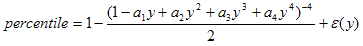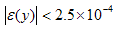# F-distribution

This algorithm (program in Matlab) calculates percentile values for given values on an F-distribution curve.

 You must provide the value of F, the degrees of freedom in the numerator and the degrees of freedom in the denominator. This Matlab code does not use any special toolbox, it uses only standard built-in functions.The F-distribution curve

The area of the shaded region represents the percentile. The tail-end value (the area of the unshaded region) is also calculated.

The F-distribution function is approximated using the following formula:where,

a1 = 0.196854
a2 = 0.115194
a3 = 0.000344
a4 = 0.019527d1 = degrees of freedom in numerator
d2 = degrees of freedom in denominatorThis is the Matlab script to perform the task:

clc; clear

% Asks the user for the relevant input
f = input ('Enter F-value: ');
d1 = input(
'Enter degrees of freedom in numerator: ');
d2 = input(
'Enter degrees of freedom in denominator: ');

x = 1;
% Computes using inverse for small F-values
if f < 1
s = d2;
t = d1;
z = 1/f;

else
s = d1;
t = d2;
z = f;

end
j = 2/(9*s);
k = 2/(9*t);

% Uses approximation formulas
y = abs((1 - k)*z^(1/3) - 1 + j)/sqrt(k*z^(2/3) + j);
if t < 4
y = y*(1 + 0.08*y^4/t^3);

end

a1 = 0.196854;
a2 = 0.115194;
a3 = 0.000344;
a4 = 0.019527;
x = 0.5/(1 + y*(a1 + y*(a2 + y*(a3 + y*a4))))^4;
x = floor(x*10000 + 0.5)/10000;

% Adjusts if inverse was computed
if f < 1
x = 1 - x;

end

% Displays results
str1 = ['Percentile = ' num2str(1-x)];
str2 = [
'Tail end value = ' num2str(x)];
disp(str1)
disp(str2)

### Example 1 - Calculate a percentile

What is the percentile on an F-distribution curve when the F-value is 0.474 and the degrees of freedom are 1 and 18?

We run the code above and enter the data:

Enter F-value: .474
Enter degrees of freedom in numerator: 1
Enter degrees of freedom in denominator: 18

Matlab response is:

Percentile = 0.4937
Tail end value = 0.5063

### Example 2

What is the percentile the F-value is 23.7 and the degrees of freedom are 3 and 6?

We run the code above and enter the data:

Enter F-value: 23.7
Enter degrees of freedom in numerator: 3
Enter degrees of freedom in denominator: 6

Matlab response is:

Percentile = 0.9984
Tail end value = 0.0016

From 'F-distribution' to Matlab home

From 'F-distribution' to 'Probability and Stats'